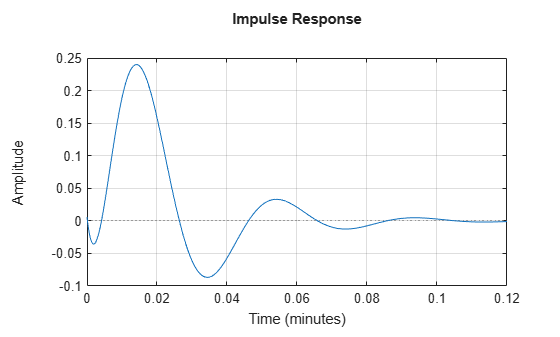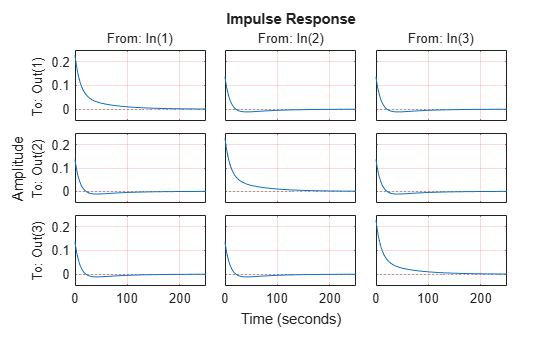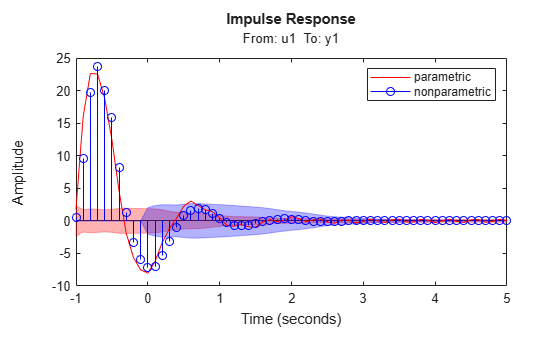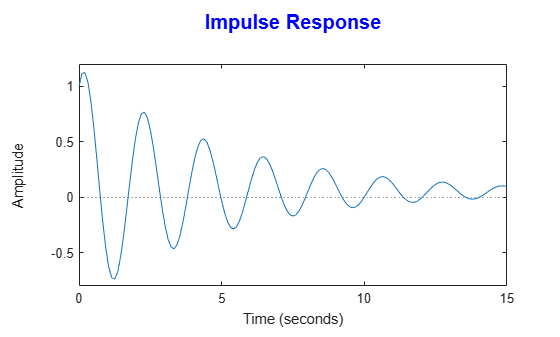# impulseplot

Plot impulse response with additional plot customization options

## Syntax

``h = impulseplot(sys)``
``h = impulseplot(sys1,sys2,...,sysN)``
``h = impulseplot(sys1,LineSpec1,...,sysN,LineSpecN)``
``h = impulseplot(___,tFinal)``
``h = impulseplot(___,t)``
``h = impulseplot(AX,___)``
``h = impulseplot(___,plotoptions)``

## Description

`impulseplot` lets you plot dynamic system impulse responses with a broader range of plot customization options than `impulse`. You can use `impulseplot` to obtain the plot handle and use it to customize the plot, such as modify the axes labels, limits and units. You can also use `impulseplot` to draw an impulse response plot on an existing set of axes represented by an axes handle. To customize an existing impulse plot using the plot handle:

1. Obtain the plot handle

2. Use `getoptions` to obtain the option set

3. Update the plot using `setoptions` to modify the required options

For more information, see Customizing Response Plots from the Command Line. To create impulse plots with default options or to extract impulse response data, use `impulse`.

example

````h = impulseplot(sys)` plots the impulse response of the dynamic system model `sys` and returns the plot handle `h` to the plot. You can use this handle `h` to customize the plot with the `getoptions` and `setoptions` commands.```

example

````h = impulseplot(sys1,sys2,...,sysN)` plots the impulse response of multiple dynamic systems `sys1,sys2,…,sysN` on the same plot. All systems must have the same number of inputs and outputs to use this syntax.```

example

````h = impulseplot(sys1,LineSpec1,...,sysN,LineSpecN)` sets the line style, marker type, and color for the impulse response of each system. All systems must have the same number of inputs and outputs to use this syntax.```

example

````h = impulseplot(___,tFinal)` simulates the impulse response from `t = 0` to the final time ```t = tFinal```. Specify `tFinal` in the system time units, specified in the `TimeUnit` property of `sys`. For discrete-time systems with unspecified sample time `(Ts = -1)`, `impulseplot` interprets `tFinal` as the number of sampling intervals to simulate.```

example

````h = impulseplot(___,t)` simulates the impulse response using the time vector `t`. Specify `t` in the system time units, specified in the `TimeUnit` property of `sys`.```
````h = impulseplot(AX,___)` plots the impulse response on the `Axes` object in the current figure with the handle `AX`.```

example

````h = impulseplot(___,plotoptions)` plots the impulse response with the options set specified in `plotoptions`. You can use these options to customize the impulse plot appearance using the command line. Settings you specify in `plotoptions` overrides the preference settings in the MATLAB® session in which you run `impulseplot`. Therefore, this syntax is useful when you want to write a script to generate multiple plots that look the same regardless of the local preferences.```

## Examples

collapse all

For this example, use the plot handle to change the time units to minutes and turn on the grid.

Generate a random state-space model with 5 states and create the impulse response plot with plot handle `h`.

```rng("default") sys = rss(5); h = impulseplot(sys);```Change the time units to minutes and turn on the grid. To do so, edit properties of the plot handle, `h` using `setoptions`.

`setoptions(h,'TimeUnits','minutes','Grid','on');`The impulse plot automatically updates when you call `setoptions`.

Alternatively, you can also use the `timeoptions` command to specify the required plot options. First, create an options set based on the toolbox preferences.

`plotoptions = timeoptions('cstprefs');`

Change properties of the options set by setting the time units to minutes and enabling the grid.

```plotoptions.TimeUnits = 'minutes'; plotoptions.Grid = 'on'; impulseplot(sys,plotoptions);```You can use the same option set to create multiple impulse plots with the same customization. Depending on your own toolbox preferences, the plot you obtain might look different from this plot. Only the properties that you set explicitly, in this example `TimeUnits` and `Grid`, override the toolbox preferences.

For this example, consider a MIMO state-space model with 3 inputs, 3 outputs and 3 states. Create a impulse plot with red colored grid lines.

Create the MIMO state-space model `sys_mimo`.

```J = [8 -3 -3; -3 8 -3; -3 -3 8]; F = 0.2*eye(3); A = -J\F; B = inv(J); C = eye(3); D = 0; sys_mimo = ss(A,B,C,D); size(sys_mimo)```
```State-space model with 3 outputs, 3 inputs, and 3 states. ```

Create an impulse plot with plot handle `h` and use `getoptions` for a list of the options available.

`h = impulseplot(sys_mimo)````h = resppack.timeplot ```
`p = getoptions(h)`
```p = Normalize: 'off' SettleTimeThreshold: 0.0200 RiseTimeLimits: [0.1000 0.9000] TimeUnits: 'seconds' ConfidenceRegionNumberSD: 1 IOGrouping: 'none' InputLabels: [1x1 struct] OutputLabels: [1x1 struct] InputVisible: {3x1 cell} OutputVisible: {3x1 cell} Title: [1x1 struct] XLabel: [1x1 struct] YLabel: [1x1 struct] TickLabel: [1x1 struct] Grid: 'off' GridColor: [0.1500 0.1500 0.1500] XLim: {3x1 cell} YLim: {3x1 cell} XLimMode: {3x1 cell} YLimMode: {3x1 cell} ```

Use `setoptions` to update the plot with the required customization.

`setoptions(h,'Grid','on','GridColor',[1 0 0]);`The impulse plot automatically updates when you call `setoptions`. For MIMO models, `impulseplot` produces a grid of plots, each plot displaying the impulse response of one I/O pair.

Compare the impulse response of a parametric identified model to a nonparametric (empirical) model, and view their 3-σ confidence regions. (Identified models require System Identification Toolbox™ software.)

Identify a parametric and a nonparametric model from sample data.

```load iddata1 z1 sys1 = ssest(z1,4); sys2 = impulseest(z1);```

Plot the impulse responses of both identified models. Use the plot handle to display the 3-σ confidence regions.

```t = -1:0.1:5; h = impulseplot(sys1,'r',sys2,'b',t); showConfidence(h,3) legend('parametric','nonparametric')```The nonparametric model `sys2` shows higher uncertainty.

For this example, examine the impulse response of the following zero-pole-gain model and limit the impulse plot to `tFinal` = 15 s. Use 15-point blue text for the title. This plot should look the same, regardless of the preferences of the MATLAB session in which it is generated.

```sys = zpk(-1,[-0.2+3j,-0.2-3j],1)*tf([1 1],[1 0.05]); tFinal = 15;```

First, create a default options set using `timeoptions`.

`plotoptions = timeoptions;`

Next change the required properties of the options set `plotoptions`.

```plotoptions.Title.FontSize = 15; plotoptions.Title.Color = [0 0 1];```

Now, create the impulse response plot using the options set `plotoptions`.

`h = impulseplot(sys,tFinal,plotoptions);`Because `plotoptions` begins with a fixed set of options, the plot result is independent of the toolbox preferences of the MATLAB session.

## Input Arguments

collapse all

Dynamic system, specified as a SISO or MIMO dynamic system model or array of dynamic system models. Dynamic systems that you can use include:

If `sys` is an array of models, the function plots the impulse response of all models in the array on the same axes.

Line style, marker, and color, specified as a character vector or string containing symbols. The symbols can appear in any order. You do not need to specify all three characteristics (line style, marker, and color). For example, if you omit the line style and specify the marker, then the plot shows only the marker and no line.

Example: `'--or'` is a red dashed line with circle markers

Line StyleDescription
`-`Solid line
`--`Dashed line
`:`Dotted line
`-.`Dash-dot line
MarkerDescription
`'o'`Circle
`'+'`Plus sign
`'*'`Asterisk
`'.'`Point
`'x'`Cross
`'_'`Horizontal line
`'|'`Vertical line
`'s'`Square
`'d'`Diamond
`'^'`Upward-pointing triangle
`'v'`Downward-pointing triangle
`'>'`Right-pointing triangle
`'<'`Left-pointing triangle
`'p'`Pentagram
`'h'`Hexagram
ColorDescription

`y`

yellow

`m`

magenta

`c`

cyan

`r`

red

`g`

green

`b`

blue

`w`

white

`k`

black

Final time for impulse response computation, specified as a scalar. Specify `tFinal` in the system time units, specified in the `TimeUnit` property of `sys`. For discrete-time systems with unspecified sample time `(Ts = -1)`, `impulseplot` interprets `tFinal` as the number of sampling intervals to simulate.

Time for impulse response simulation, specified as a vector. Specify the time vector `t` in the system time units, specified in the `TimeUnit` property of `sys`. The time vector must be real, finite, and must contain monotonically increasing and evenly spaced time samples.

The time vector `t` is:

• t = Tinitial:Tsample:Tfinal, for discrete-time systems.

• t = Tinitial:dt:Tfinal, for continuous-time systems. Here, dt is the sample time of a discrete approximation of the continuous-time system.

Target axes, specified as an `Axes` object. If you do not specify the axes and if the current axes are Cartesian axes, then `impulseplot` plots on the current axes. Use `AX` to plot into specific axes when creating a impulse plot.

Impulse plot options set, specified as a `TimePlotOptions` object. You can use this option set to customize the impulse plot appearance. Use `timeoptions` to create the option set. Settings you specify in `plotoptions` overrides the preference settings in the MATLAB session in which you run `impulseplot`. Therefore, `plotoptions` is useful when you want to write a script to generate multiple plots that look the same regardless of the local preferences.

For the list of available options, see `timeoptions`.

## Output Arguments

collapse all

Plot handle, returned as a `handle` object. Use the handle `h` to get and set the properties of the impulse plot using `getoptions` and `setoptions`. For the list of available options, see the Properties and Values Reference section in Customizing Response Plots from the Command Line.

## Version History

Introduced before R2006a